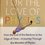# Draw me until you match me

I want nobody to tell the correct answer here.I already bought the discussion and the answer seems wrong to me. I just wanted to know what is wrong with this approach:

First, let us calculate total number of such possible ways ,

Case-1 : We get the same numbers in first and second turns,

For the first turn, we have 4 options and for the second turn , we have only one option, hence total number of ways = $4$.

Case-2 : First 2 are different and the third is same as either first or second.

Hence, first turn has 4 options, second turn has 3 options and third turn has 2 options(either same as first turn or same as second turn). Hence no. of ways = $24$.

Case-3 : First three numbers are different and fourth one is same as either of them.

First turn has 4, second turn has 3, third turn has 2 and fourth turn has 3 options, hence $72$ ways.

Case-4 : First four turns are different and the fifth turn is either of them.(Note that fifth turn has 4 options).

Number of ways = $4! \times 4$ = $96$

Hence total number of ways = $196$

Now, let us find no. of favourable ways. Note that now, in every case last turn will have only $1$ option, as it is same as the first one. Hence everytime i will be multiplying by 1 showing last turn has 1 option only.

Case - 1: First 2 turns are same. $4$ ways.(every possible way will satisfy)

Case-2 : First 2 turns are different and third turn is same as first.

$4 \times 3 \times 1 = 12$ ways

Case - 3: First 3 turns are different and fourth one is same as the first one :

$4 \times 3 \times 2 \times 1 = 24$ ways.

Case-4 : First four turns are different and fifth turn is same as first turn :

$4 \times 3 \times 2 \times 1 \times 1 = 24$ ways.

Hence total number of favorable ways = $64$.

Hence probability = $\frac{64}{196} = \boxed{\frac{16}{49}} .$6 years, 7 months ago

This discussion board is a place to discuss our Daily Challenges and the math and science related to those challenges. Explanations are more than just a solution — they should explain the steps and thinking strategies that you used to obtain the solution. Comments should further the discussion of math and science.

When posting on Brilliant:

• Use the emojis to react to an explanation, whether you're congratulating a job well done , or just really confused .
• Ask specific questions about the challenge or the steps in somebody's explanation. Well-posed questions can add a lot to the discussion, but posting "I don't understand!" doesn't help anyone.
• Try to contribute something new to the discussion, whether it is an extension, generalization or other idea related to the challenge.

MarkdownAppears as
*italics* or _italics_ italics
**bold** or __bold__ bold
- bulleted- list
• bulleted
• list
1. numbered2. list
1. numbered
2. list
Note: you must add a full line of space before and after lists for them to show up correctly
paragraph 1paragraph 2

paragraph 1

paragraph 2

[example link](https://brilliant.org)example link
> This is a quote
This is a quote
    # I indented these lines
# 4 spaces, and now they show
# up as a code block.

print "hello world"
# I indented these lines
# 4 spaces, and now they show
# up as a code block.

print "hello world"
MathAppears as
Remember to wrap math in $$ ... $$ or $ ... $ to ensure proper formatting.
2 \times 3 $2 \times 3$
2^{34} $2^{34}$
a_{i-1} $a_{i-1}$
\frac{2}{3} $\frac{2}{3}$
\sqrt{2} $\sqrt{2}$
\sum_{i=1}^3 $\sum_{i=1}^3$
\sin \theta $\sin \theta$
\boxed{123} $\boxed{123}$

Sort by:

Each way you list in Case 1 is four times more probable than each way you list in Case 2. Similar with other things. They are not equiprobable.

- 6 years, 7 months ago